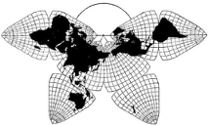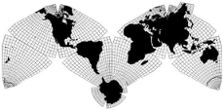Cahill 1909 Go back to Gene Keyes home pageCahill-Keyes 1975

Page 4 of 6
 Cahill-Keyes Octant Graticule: Principles and Specifications with Perl programs and OpenOffice.org 2.0 macros for 1/1,000,000 Megamap Gene Keyes 2010-08-20

 Continued from page 3 11) Perl program "OOmacroMaker4" by Mary Jo Graça to produce OpenOffice.org Draw macros for Cahill-Keyes Octant Graticule Notes by Gene Keyes and Mary Jo Graça This second of two Perl programs by Mary Jo Graça reads the .csv file output by her previous Perl program "HalfOctant8", and prepares a file with OOo macros to draw the scaffold half triangle, the half octant, minor parallels, minor meridians, major parallels, and major meridians. (Major = bolded at 5° intervals.) Macros in the same conglomerate assemble the half octants into a full 8-octant world map profile, and draw a grid of systemic intervals (p. 6). Future macros may (hopefully) adapt GIS data for coastlines and borders.

 `#! /usr/bin/perl -w# Program OOmacroMaker4. This program reads the .csv file output by program# HalfOctant8, and prepares a file with OOo macros to draw the scaffold half triangle,# the half octant, minor parallels, minor meridians, major parallels, and major meridians.## The content of the output file will have to be copied into a macro of an OpenOffice.org# Draw file. It may have to be copied some chunks at a time, because the copy function# might not be able to handle the many lines.# The macros require functions L, P, Z and A, which this program does not write.# # NOTE: There is only one single file written; each macro is written as a sub, to that file;# - while preparing each of those subs, they reside in string variables.## - all the coordinates and angles are multiplied by 100 and given as integers, so that they# are units in 1/100 of a mm or of a degree, of type Long, as OOo Basic wants# - all the y-values are given as the negative of what they were, to take into account that# OOo has y positive downwards, whereas my definition is y positive upwards.## NOTE 2: When running the program, there are messages that variables L, dP, and Length# are used only once. There is no problem; these hashes are read but are not needed for# the macros.# Read in all the arrays output by program HalfOctant8.# Names of arrays are in sub ReadCSV. I am using arrays of arrays for the hashes.open (CSV, "OOMacros8");\$Skip = "";unless (\$Skip) { # Prepare OO macro to draw octant \$Mac = "Sub HalfOctant\nD=ThisComponent\nG=D.DrawPages(0)\n"; \$Mac .= "S=L(D,G,RGB(102,0,153))\nN=Array(" . P(\$xOctant,\$yOctant); \$n = @xOctant - 1; foreach \$i (1..\$n) { \$Mac .= ",_\n" . P(\$xOctant[\$i],\$yOctant[\$i]); } \$Mac .= " )\nS.PolyPolygon=Array(N)\nEnd Sub\n\n"; print OO \$Mac;} # End skip of preparing OO macro to draw octant\$Skip = "";unless (\$Skip) { # Prepare OO macro to draw triangle, in light grey \$n = @xTriangle - 1; \$Mac = "Sub Triangle\nD=ThisComponent\nG=D.DrawPages(0)\n"; # Start with last point, and then add all points, so that last point is used twice; this # creates a closed shape without filling it with color \$Mac .= "S=L(D,G,RGB(200,200,200))\nN=Array(" . P(\$xTriangle[\$n],\$yTriangle[\$n]); foreach \$i (0..\$n) { \$Mac .= ",_\n" . P(\$xTriangle[\$i],\$yTriangle[\$i]); } \$Mac .= " )\nS.PolyPolygon=Array(N)\nEnd Sub\n\n"; print OO \$Mac;} # End skip of preparing OO macro to draw triangle\$Skip = "";unless (\$Skip) { # Prepare OO macro to draw meridians \$Major = "Sub MeridiansMajor\nD=ThisComponent\nG=D.DrawPages(0)\n"; \$Minor = "Sub MeridiansMinor\nD=ThisComponent\nG=D.DrawPages(0)\n"; \$Major .= "C=RGB(0,0,255)\n"; \$Minor .= "C=RGB(102,204,204)\n"; foreach \$m (0..44) { # For each meridian, except 45°, which is octant boundary # Will later add line to draw object in correct color to before \$Mac; # \$Mac is just temporary for a single meridian. \$Mac = "N=Array(" . P(\$xJ[\$m],\$yJ[\$m]); foreach \$j (1..3) { # Polar start, frigid joint, tropic joint, and equator points \$Mac .= ",_\n" . P(\$xJ[\$m][\$j],\$yJ[\$m][\$j]); } \$Mac .= " )\nS.PolyPolygon = Array(N)\n"; if (\$m % 5 == 0) { # Major meridians \$Major .= "S=L(D,G,C)\n" . \$Mac; } else { # Minor meridians \$Minor .= "S=L(D,G,C)\n" . \$Mac; } } print OO \$Major,"End Sub\n\n"; print OO \$Minor,"End Sub\n\n";} # End of skipping the macros for the meridians\$Skip = "";unless (\$Skip) { # Prepare OO macro to draw parallels \$Major = "Sub ParallelsMajor\nD=ThisComponent\nG=D.DrawPages(0)\n"; \$Minor = "Sub ParallelsMinor\nD=ThisComponent\nG=D.DrawPages(0)\n"; \$Major .= "C=RGB(0,0,255)\n"; \$Minor .= "C=RGB(102,204,204)\n"; # Torrid and Temperate zones# foreach \$p (1..14,16..72) { # For each parallel, except 0°, which is octant boundary# Note: not drawing parallel 15° as partially an arc; if we want an arc, comment the# following line, uncomment the preceding one, and delete Yes in the next Skip= # statement. foreach \$p (1..72) { # For each parallel, except 0°, which is octant boundary # Will later add line to draw object in correct color to before \$Mac; # \$Mac is just temporary for a single parallel or parallel object. \$Mac = "N=Array(" . P(\$xP[\$p],\$yP[\$p]); foreach \$m (1..45) { # For each node at a meridian \$Mac .= ",_\n" . P(\$xP[\$m][\$p],\$yP[\$m][\$p]); } \$Mac .= ")\nS.PolyPolygon=Array(N)\n"; if (\$p % 5 == 0) { # Major \$Major .= "\'P \$p\nS=L(D,G,C)\n" . \$Mac; } else { # Minor \$Minor .= "\'P \$p\nS=L(D,G,C)\n" . \$Mac; } }# Note: if we want to do the arc for parallel 15°, delete the word Yes, here, and on the# foreach loop above use the foreach that skips parallel 15°.\$Skip = "Yes";unless (\$Skip) { # Parallel 15°. # Segmented line portion \$Mac = "N=Array(" . P(\$xP,\$yP); foreach \$m (1..30) { # For each node at a meridian \$Mac .= ",_\n" . P(\$xP[\$m],\$yP[\$m]); } \$Mac .= " )\nS.PolyPolygon=Array(N)\n"; \$Major .= "\'P 15\nS=L(D,G,C)\n" . \$Mac; # Circular arc section of Parallel 15° use Math::Trig; \$R = sqrt((\$xP - \$xC)**2 + (\$yP - \$yC)**2); \$Start = rad2deg(atan2(\$yP - \$yC, \$xP - \$xC) ); \$End = rad2deg(atan2(\$yP - \$yC, \$xP - \$xC) ); \$Mac = sprintf ("%.0f,%.0f,%.0f,%.0f,%.0f", \$xC*100,-\$yC*100,\$R*100,\$Start*100,\$End*100); \$Major .= "G.add(A(D,\$Mac,C))\n";} # End skip of circular arc section of Parallel 15° # Frigid supple zone # Straight line of parallel 73° -- single straight line, but using PolyLine, anyway \$Mac = "\'P 73\nS=L(D,G,C)\n"; \$Mac .= "N=Array(" . P(\$xP,\$yP) . "," . P(\$xP,\$yP); \$Mac .= ")\nS.PolyPolygon=Array(N)\n"; \$Minor .= \$Mac; # Segmented-line section of parallel 74° \$Mac = "\'P 74\nS=L(D,G,C)\n N=Array(" . P(\$xP,\$yP); foreach \$m (31..45) { # For each node at a meridian \$Mac .= ",_\n" . P(\$xP[\$m],\$yP[\$m]); } \$Mac .= ")\nS.PolyPolygon=Array(N)\n"; \$Minor .= \$Mac; # Arcs of parallels 73° and 74° for \$p (73..74) { \$R = \$xP[\$p] - \$xA; \$Mac = sprintf ("%.0f,%.0f,%.0f",\$xA*100,-\$yA*100,\$R*100); \$Minor .= "\'P \$p\nG.add(A(D,\$Mac,0,3000,C))\n"; } # Frigid zone for \$p (75..89) { \$R = \$xP[\$p] - \$xA; \$Mac = sprintf ("%.0f,%.0f,%.0f",\$xA*100,-\$yA*100,\$R*100); if (\$p % 5 == 0) { # Major \$Major .= "\'P \$p\nG.add(A(D,\$Mac,0,4500,C))\n"; } else { # Minor \$Minor .= "\'P \$p\nG.add(A(D,\$Mac,0,4500,C))\n"; } } print OO \$Major,"End Sub\n\n"; print OO \$Minor,"End Sub\n\n";} # End of skipping of doing parallelsclose(OO);# - - - - - - - - - - - - - - - - - - - - - - - - - - - - - - - - - - - - - - - - - - - - - -sub P { # Takes in two values, multiplies them by 100, rounds them to an integer, # multiplies the second one by -1, and returns string: P(number,number) # E.g. input 23.546,23.546 would return "P(2355,-2355)" return sprintf("P(%.0f,%.0f)", \$_*100, -\$_*100);} # end of sub Psub ReadCSV { # It is assumed that the file is already opened, with filehandle CSV while () { \$Line = \$_; chomp (\$Line); if (\$Line eq "") { next; # blank line } elsif (index(\$Line, "dP") >= 0) { @dP = ReadArray(); } elsif (index(\$Line,"L") >= 0) { @L = ReadArray(); } elsif (index(\$Line,"xJ") >=0) { @xJ = ReadArray(); } elsif (index(\$Line,"yJ") >=0) { @yJ = ReadArray(); } elsif (index(\$Line,"xP") >=0) { @xP = ReadArray(); } elsif (index(\$Line,"yP") >=0) { @yP = ReadArray(); } elsif (index(\$Line,"Points") >=0) { # Format is slightly different for this line/array/hash \$Line = ; chomp (\$Line); (\$i, @xOctant[0,1,2,3,4,5], @xTriangle[1,2],\$xC) = split(/\t/,\$Line); \$Line = ; chomp (\$Line); (\$i, @yOctant[0,1,2,3,4,5], @yTriangle[1,2],\$yC) = split(/\t/,\$Line); \$xTriangle  = \$xOctant ; # Point G \$yTriangle  = \$yOctant ; # Point G } elsif (index(\$Line,"Lengths") >=0) { # Format is slightly different for this array (\$i, @LengthNames) = split(/\t/,\$Line); \$Line = ; chomp (\$Line); (\$i, @temp) = split(/\t/,\$Line); \$n = @temp - 1; foreach \$i (0..\$n) { \$Length{\$LengthNames[\$i]} = \$temp[\$i]; } } else { print "Don\'t know what to do with this line:\n"; print \$Line, "\n"; } # End of if-statement } # End of reading data} # End of sub ReadCSVsub ReadArray { my (\$Line, \$i, \$j, @temp, @Array); while () { \$Line = \$_; chomp (\$Line); if (\$Line eq "") {last;} # blank line means end of this array (\$i, @temp) = split(/\t/,\$Line); \$n = @temp - 1; if (\$i eq "") {next;} # Ignore header line foreach \$j (0 .. \$n) { \$Array [\$j][\$i] = \$temp[\$j];} # save values } return @Array;} #End of sub ReadArray`

 On the next page is the text of the actual OOo composite macros aided by this Perl program.

Go to page 5, OpenOffice.org (OOo) macros via Perl

Go back to page 1     Go back to page 2     Go back to page 3
Go back to B.J.S. Cahill Resource Page